Function Repository Resource:

# ReflectPoints

Reflect a set of points over a line, plane or hyperplane defined by points

Contributed by: Ed Pegg Jr and Jan Mangaldan
 ResourceFunction["ReflectPoints"][mirror,pts] reflects pts across mirror.

## Details

ResourceFunction["ReflectPoints"] works on points of any dimension.

## Examples

### Basic Examples (2)

Reflect points about a given line:In:=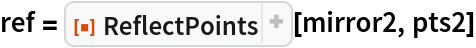Out=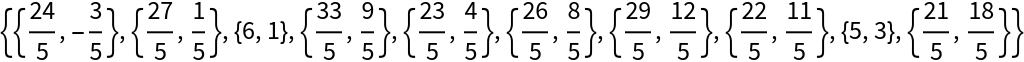In:=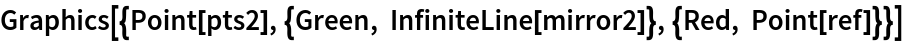Out=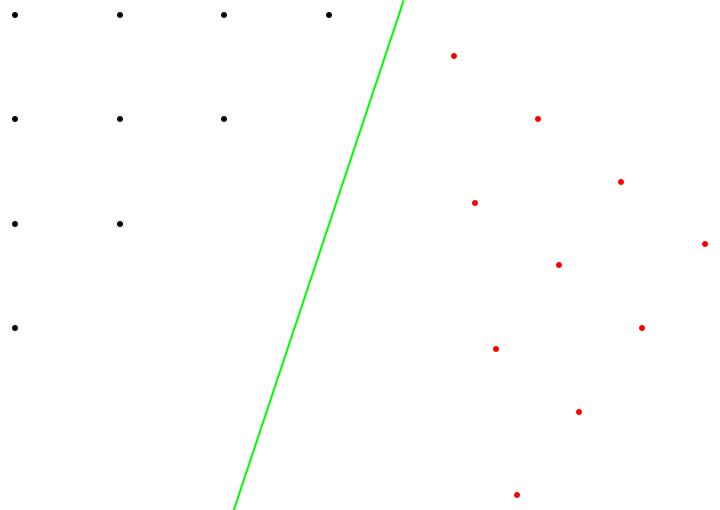Reflect points about a given plane:In:=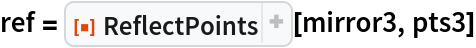Out=In:=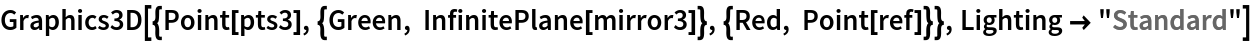Out=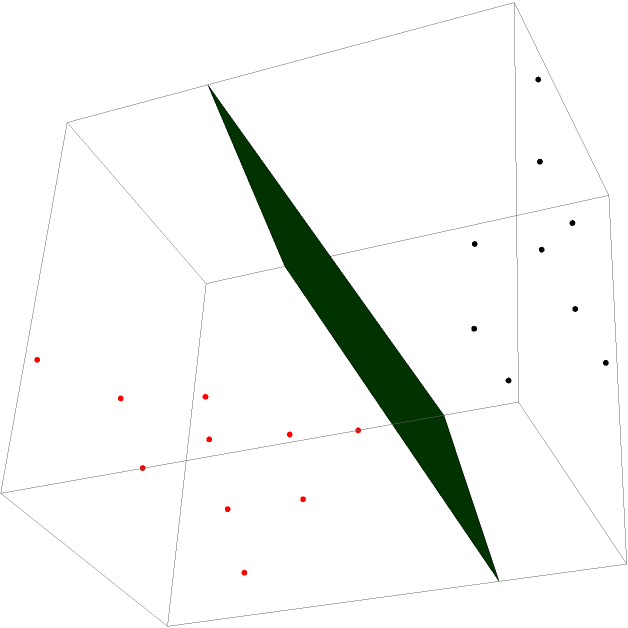### Scope (2)

Reflect a single point:

 In:=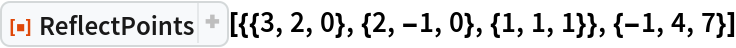Out=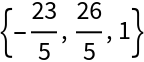Generate two sets of random 4D points, one representing a mirror, and the other representing points to transform:Reflect 4D points about a hyperplane:

 In:=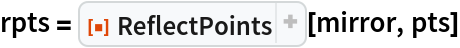Out=Construct a Hyperplane representation of the mirror:

 In:=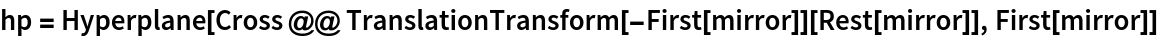Out=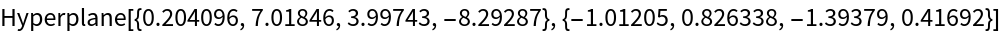Show that the distance between the points and their corresponding reflections is equal to the sum of their distances from the reflecting hyperplane:

 In:=Out=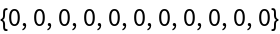## Requirements

Wolfram Language 11.3 (March 2018) or above

## Version History

• 1.1.0 – 22 August 2022
• 1.0.1 – 31 January 2022
• 1.0.0 – 11 February 2019